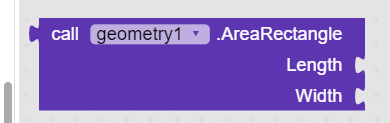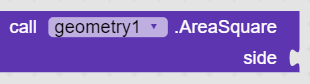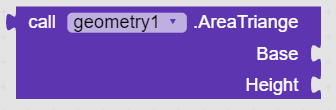# My First Extension Geometry

### Short description of my Extension

This Extension Can Give value of pi, area of circle , Diameter of circle etc.

### Description of all the blocks

Detailed Version

io.sashi.geo - Extensions Docs (000webhostapp.com)## AreaOfCircle

Area Of Circle. In this block there are 1 parameters,## AreaRectangle

Area of a Rectangle. In this block there are 2 parameters,
Length number Width numberAreaSquare
Area of a Square. In this block there are 1 parameters,
side number## AreaTriange

Area of a Right angled Triangale. In this block there are 2 parameters,
Base number Height number

## Diameter_Circle

Diameter Circle. In this block there are 1 parameters,
METHODType - Return number

## PerimenteTriangle

Primeter of a Triangale. In this block there are 1 parameters,
Side number
METHODType - Return number

## PerimeterPolygon

Perimeter of a Polygon. In this block there are 2 parameters,
Side_Length number No_Of_Sides number
METHODType - Return number

## PerimeterRectangle

Perimeter of a Rectangle. In this block there are 2 parameters,
Length number Width number
METHODType - Return number

## PerimeterSquare

Perimeter of a Square. In this block there are 1 parameters,
Side number
METHODType - Return number

## Pi

mathematical constant ?. In this block there are 0 parameters,